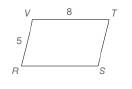Chapter 4.2, Problem 2EElementary Geometry For College St...

7th Edition
Alexander + 2 others
ISBN: 9781337614085

Solutions

Chapter
SectionElementary Geometry For College St...

7th Edition
Alexander + 2 others
ISBN: 9781337614085
Textbook Problem

a) As shown, must R S T V be a parallelogram?   b ) With measures as shown, is it necessary that R S = 8 ?To determine

a)

Whether RSTV a parallelogram.

Explanation

The opposite sides of a parallelogram are equal and parallel.

Calculation:

Since, the opposite sides of the shown figure RSTV is equal and parallel RSTV is a parallelogram...

To determine

b)

Whether it is necessary that RS=8.

Still sussing out bartleby?

Check out a sample textbook solution.

See a sample solution

The Solution to Your Study Problems

Bartleby provides explanations to thousands of textbook problems written by our experts, many with advanced degrees!

Get Started

Find more solutions based on key concepts